# numpy中三维数组中加入元素后的位置详解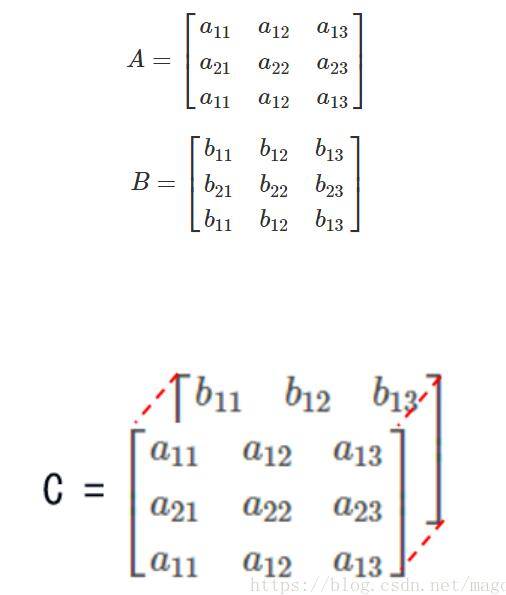``````
def test3D():
import numpy as np
data_array = np.zeros((3, 5, 6), dtype=np.int)
data_array[1, 2, 2] = 1

print(data_array)``````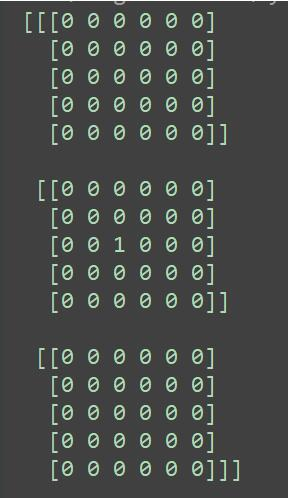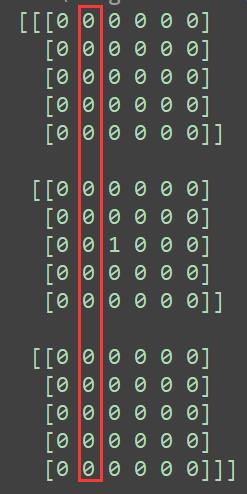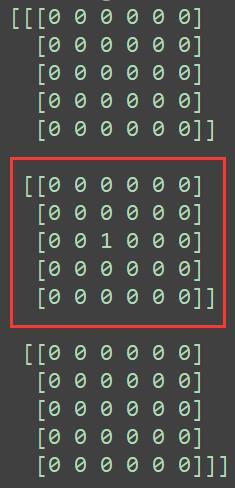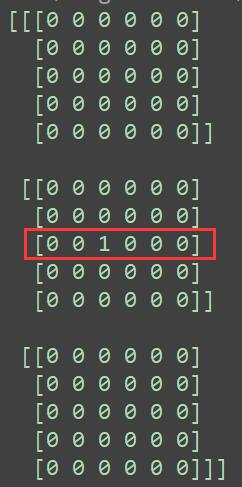``````
>>> np.random.seed(123)
>>> X = np.random.randint(0, 5, [3, 2, 2])
>>> print(X)

[[[5 2]
[4 2]]

[[1 3]
[2 3]]

[[1 1]
[0 1]]]

>>> X.sum(axis=0)
array([[7, 6],
[6, 6]])

>>> X.sum(axis=1)
array([[9, 4],
[3, 6],
[1, 2]])

>>> X.sum(axis=2)
array([[7, 6],
[4, 5],
[2, 1]])``````

numpy 三维数组 位置